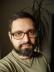## Product SubArray

Given a list of 4 or more numbers, write a script to find the contiguous sublist that has the maximum product. The length of the sublist is irrelevant; your job is to maximize the product.

Example

Input: `[ 2, 5, -1, 3 ]`

Output: `[ 2, 5 ]` which gives maximum product `10`.

The easiest (but probably not the fastest) method would be to start from each position and compute the products of all the possible sublists starting at the position, remembering the positions where the product is maximal.

I automatically reached for List::Util’s `product` to get the products easily, but alas! Running

``product(-1, 3)``

caused a Floating point exception (bug reported). So, I had to implement `product` myself:

``````#! /usr/bin/perl
use warnings;
use strict;

sub product {
my @list = @_;
my \$p = 1;
\$p *= \$_ for @list;
return \$p
}

sub max_prod {
my (\$list) = @_;
my \$max = [\$list->];
for my \$i (0 .. \$#\$list) {
for my \$j (\$i .. \$#\$list) {
my \$p = product(@\$list[\$i .. \$j]);
\$max = [\$p, @\$list[\$i .. \$j]] if \$p > \$max->;
}
}
return \$max
}

use Test::More tests => 1;
is_deeply max_prod([2, 5, -1, 3]), [10, 2, 5];``````

The `max_prod` subroutine returns the product and the list in this order.

Note: Don’t confuse `max_prod` with Max Brod.

## IPv4 Partition

You are given a string containing only digits (0..9). The string should have between 4 and 12 digits.

Write a script to print every possible valid IPv4 address that can be made by partitioning the input string.

For the purpose of this challenge, a valid IPv4 address consists of four “octets” i.e. A, B, C and D, separated by dots (`.`).

Each octet must be between 0 and 255, and must not have any leading zeroes. (e.g., `0` is OK, but `01` is not.)

Example

Input: `25525511135`,

Output:

```255.255.11.135
255.255.111.35```

This problem is asking for a recursive solution: Given an already extracted previous octets, try to extract one, two, or three characters to create one more octet (if possible) and run the same subroutine on the remaining string(s).

The “if possible” means we have to check whether the prefix isn’t greater to 255, whether it doesn’t start with a 0 (unless it’s 0 itself) or whether there are enough digits to create an octet of the given length.

Once we’ve accumulated 4 octets and the string’s been exhausted, we have a solution.

``````#! /usr/bin/perl
use warnings;
use strict;

sub _partition {
my (\$string) = @_;
my @p;
for my \$pos (1 .. 3) {
next if \$pos > length \$string;

my \$prefix = substr \$string, 0, \$pos;
next if \$prefix > 255 || (
1 < length \$prefix
&& 0 == index \$prefix, '0');

if (\$pos == length \$string) {
push @p, [\$prefix];
} else {
push @p, grep @\$_ <= 4,
map [\$prefix, @\$_],
_partition(substr \$string, \$pos);
}
}
return @p
}

sub partition {
my (\$string) = @_;
[ map { join '.', @\$_ } grep 4 == @\$_, _partition(\$string) ]
}

use Test::More;
use Test::Deep;

cmp_deeply partition('25525511135'),
bag(qw( 255.255.11.135 255.255.111.35 ));

cmp_deeply partition('1234'), [ '1.2.3.4'];
cmp_deeply partition('123405'),
bag(qw( 1.23.40.5 1.234.0.5 12.3.40.5 12.34.0.5 123.4.0.5 ));

done_testing();``````I blog about Perl.